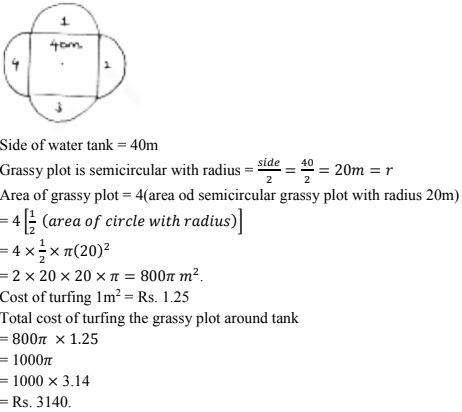# Chapter 15 Areas Related to Circles RD Sharma Solutions Exercise 15.4 Class 10 Maths

 Chapter Name RD Sharma Chapter 15 Areas Related to Circles Book Name RD Sharma Mathematics for Class 10 Other Exercises Exercise 15.1Exercise 15.2Exercise 15.3 Related Study NCERT Solutions for Class 10 Maths

### Exercise 15.4 Solutions

1. A plot is in the form of rectangle ABCD having semi - circle on BC. If AB = 60m and BC = 28m, find the area of plot.

Solution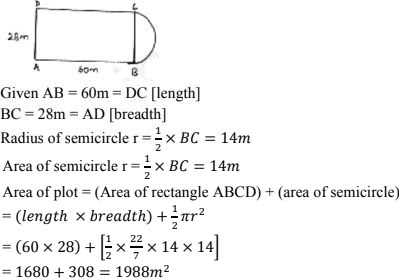2. A playground has the shape of rectangle, with two semicircles on its smaller sides as diameters, added to its outside. If the sides of rectangle are 36m and 24.5m. Find the area of playground.

Solution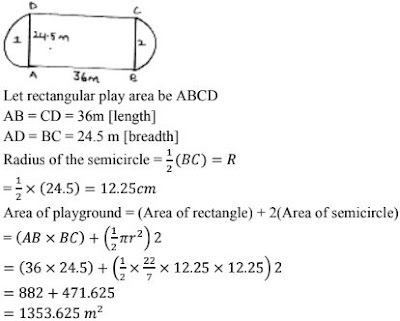3. The outer circumference of a circular race track is 528m. The track is everywhere 14m wide. Calculate the cost of leveling the track at rate of 50 paise per square metre.

Solution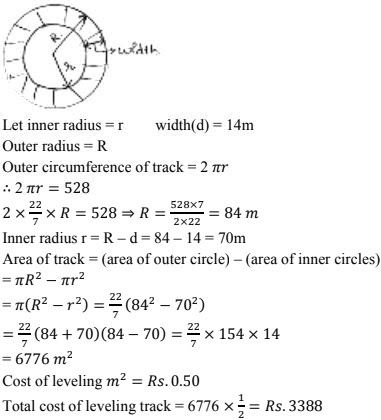4. A rectangular piece is 20m long and 15m wide from its four corners, quadrants of 3.5m radius have been cut. Find the area of remaining part.

Solution5. Four equal circles, each of radius 5 cm touch each other as shown in fig. Find the area included between them.

Solution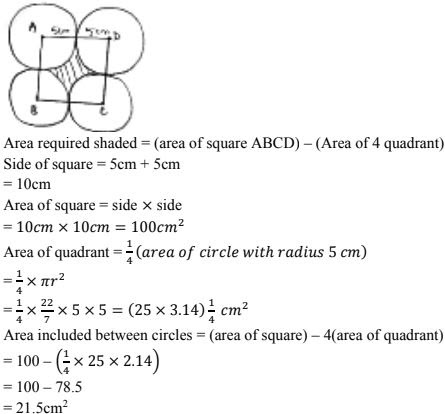6. Four cows are tethered at four corners of a square plot of side 50m, so that' they just cant reach one another. What area will be left ungrazed.

Solution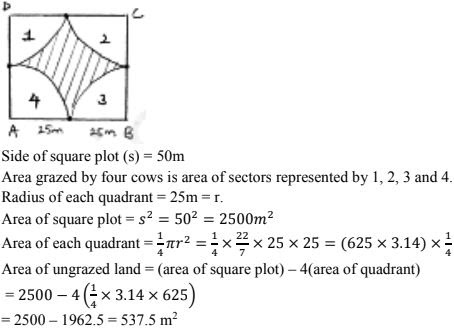7. A road which is 7m wide surrounds a circular park whose circumference is 352 m. Find the area of road.

Solution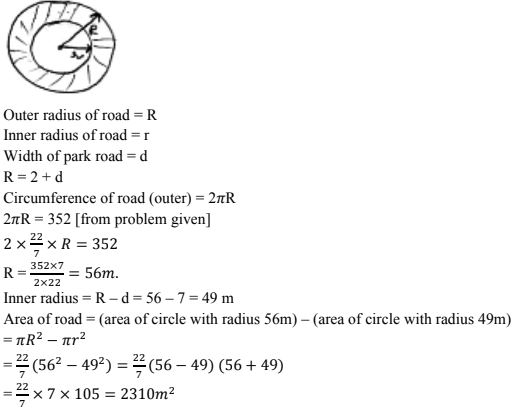8. Four equal circles each of radius a, touch each other. Show that area between them is 6/7 a2 .

Solution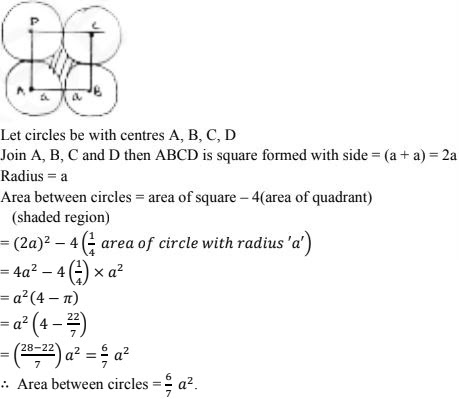9. A square water tank has its side equal to 40m, there are 4 semicircular flower beds grassy plots all around it. Find the cost of turfing the plot at Rs 1.25/sq.m.

Solution